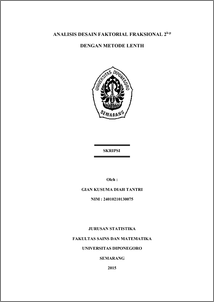# ANALISIS DESAIN FAKTORIAL FRAKSIONAL 2k-p DENGAN METODE LENTH

TANTRI, GIAN KUSUMA DIAH (2015) ANALISIS DESAIN FAKTORIAL FRAKSIONAL 2k-p DENGAN METODE LENTH. Undergraduate thesis, FSM Universitas Diponegoro.Preview
PDF
2674Kb

## Abstract

Fractional factorial design is widely used in the experiments, especially in industrial sector because it can determine the influence of main factors and interaction of response. Design involving k factor with two levels and using 2-p fraction of the full factorial experiment be called fractional factorial design 2k-p. Determination significant factor if the observed data is unreplicated can be tested using Lenth’s method. This study aims to determine the estimator and test statistics to get a significant factor with Lenth’s method, and determine the differences from Lenth’s method and classical method. The method used in the study using Lenth’s method to analyze fractional factorial unreplicated design 2k-p. Suppose that used 25-1 fractional factorial design with A, B, C, D, E factors. Based on test results obtained with the Lenth’s method estimated value of S0 and σ ̂_PSE as estimator. Margin Error and Simultaneous Margin Error value as a margin of error to determined of significant factors. Factors that influence the response are B and C. When tested with classical methods derived factors that affect the response are B, C, D, E, AB, AC, and BC, so it can be said that the classical method is more sensitive than the Lenth’s method. Keywords: Factorial, fractional, unreplicated factorial, normal probability plots, Lenth’s method

Item Type: Thesis (Undergraduate) H Social Sciences > HA Statistics Faculty of Science and Mathematics > Department of Statistics 47202 Mr Hasbi Yasin 29 Dec 2015 09:47 29 Dec 2015 09:47

Repository Staff Only: item control page